tensorflow 基礎教學

# Activation Functions 激勵函數

#### 4. 計算量節省

softmax這個常用於最後一個激勵函數

softmax：值介於 [0,1] 之間，且機率總和等於 1，適合多分類使用。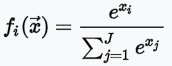```import math
z = [1.0, 2.0, 3.0, 4.0, 1.0, 2.0, 3.0]
z_exp = [math.exp(i) for i in z]
print(z_exp)  # Result: [2.72, 7.39, 20.09, 54.6, 2.72, 7.39, 20.09]
sum_z_exp = sum(z_exp)
print(sum_z_exp)  # Result: 114.98
softmax = [round(i / sum_z_exp, 3) for i in z_exp]
print(softmax)  # Result: [0.024, 0.064, 0.175, 0.475, 0.024, 0.064, 0.175]
```
1. sigmoid：值介於 [0,1] 之間，且分布兩極化，大部分不是 0，就是 1，適合二分法。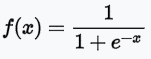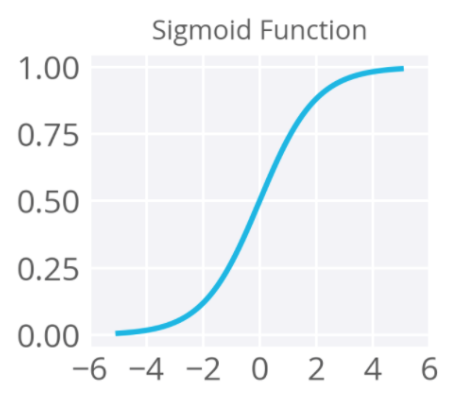2. Relu (Rectified Linear Units)：忽略負值，介於 [0,∞] 之間。3. tanh：與sigmoid類似，但值介於[-1,1]之間，即傳導有負值。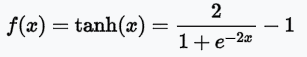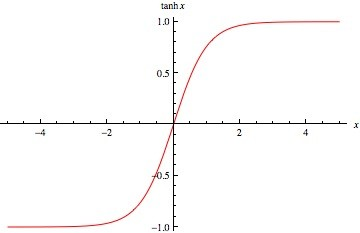#### 什麼是 One-hot encoding？

One-hot encoding 是將類別以 (0, 1) 的方式表示，舉例來說，假設有 cat、dog、bird 三個類別，而三個類別可以用 (1, 0, 0)、(0, 1, 0)、(0, 0, 1) 來表示。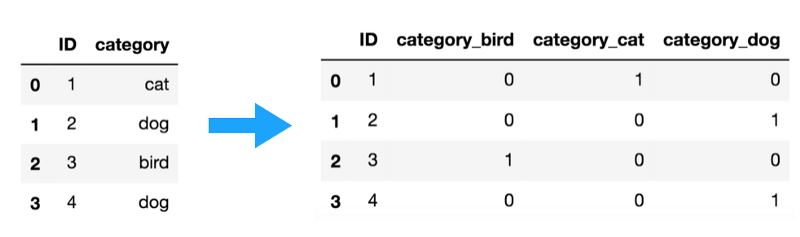Be the First to comment.